## How to analyze survey data in Excel?

If there is a list of survey data in a worksheet as shown as below, and you need to analyze this survey and generate a survey result report in Excel, how could you do? Now, I talk about the steps about analyzing survey data and generate a result report in Microsoft Excel.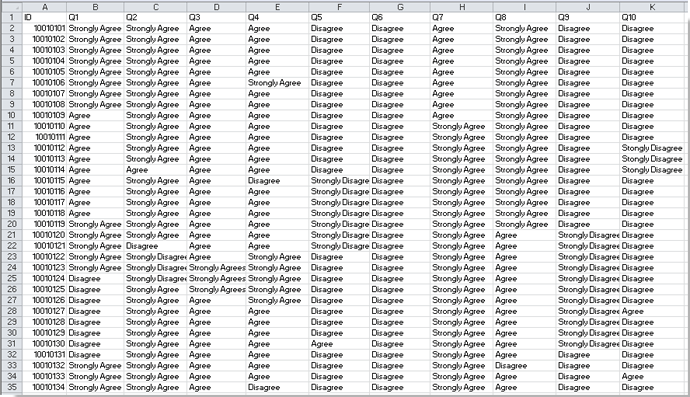#### Analyze a survey data in Excel

Part 1: Count all kinds of feedbacks in the survey

Part 2: Calculate the percentages of all feedbacks

Part 3: Generate a survey report with calculated results above

####Part 1: Count all kinds of feedbacks in the survey###### Save 50% of your time, and reduce thousands of mouse clicks for you every day!

Firstly, you need to count the total number of feedback in each question.

1. Select a blank cell, for instance, the Cell B53, type this formula =COUNTBLANK(B2:B51) (the range B2:B51 is the range of the feedback on question 1, you can change it as you need) in it, and press Enter button on the keyboard. Then drag the fill handle to the range you want to use this formula, here I fill it to the range B53: K53. See screenshot: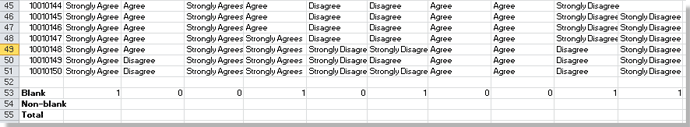2. In the Cell B54, type this formula =COUNTA(B2:B51) (the range B2:B51 is the range of the feedback on question 1, you can change it as you need) into it, and press Enter button on the keyboard. Then drag the fill handle to the range you want to use this formula, here I fill it to the range B54: K54. See screenshot: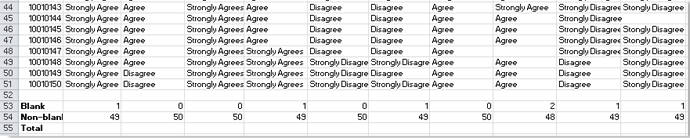3. In the Cell B55, type this formula =SUM(B53:B54) (the range B2:B51 is the range of the feedback on question 1, you can change it as you need) in to it, and press Enter button on the keyboard, then drag the fill handle to the range you want to use this formula, here I fill it to the range B55: K55. See screenshot: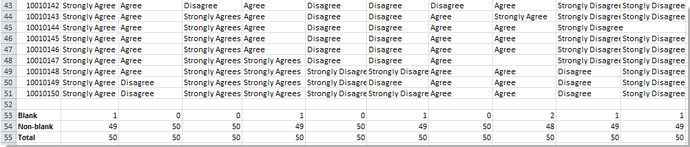Then count the number of each Strongly Agree, Agree, Disagree and Strongly Disagree on every question.

4. In the Cell B57, type this formula =COUNTIF(B2:B51,\$B\$51) (the range B2:B51 is the range of the feedback on question 1, the cell \$B\$51 is the criteria you want to count, you can change them as you need) in to it, and press Enter button on the keyboard, then drag the fill handle to the range you want to use this formula, here I fill it to the range B57: K57. See screenshot: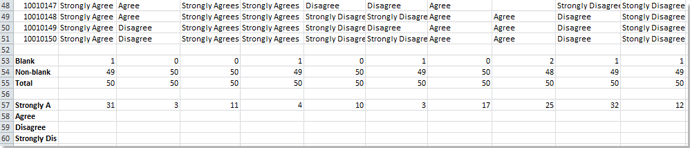5. Type =COUNTIF(B2:B51,\$B\$11) (the range B2:B51 is the range of the feedback on question 1, the cell \$B\$11 is the criteria you want to count, you can change them as you need) in to cell B58, and press Enter button on the keyboard, then drag the fill handle to the range you want to use this formula, here I fill it to the range B58: K58. See screenshot: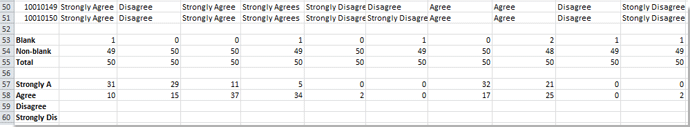6. Repeat step 5 or 6 to count the number of each feedback on the every question. See screenshot: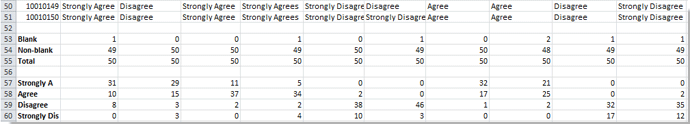7. In the Cell B61 type this formula =SUM(B57:B60) (the range B2:B51 is the range of the feedback on question 1, you can change it as you need) to sum the total feedback and press Enter button on the keyboard, then drag the fill handle to the range you want to use this formula, here I fill it to the range B61: K61. See screenshot: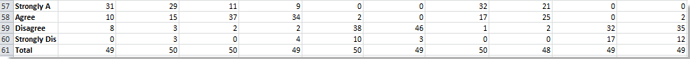####Part 2: Calculate the percentages of all feedbacks

Then you need to calculate the percentage of each feedback on every question.

8. In the Cell B62 type this formula =B57/\$B\$61 (the Cell B57 indicates the special feedback you want to count its number, the Cell \$B\$61 stands the total number of feedbacks, you can change them as you need) to sum the total feedback and press Enter button on the keyboard, then drag the fill handle to the range you want to use this formula. Then format the cell as percentage by right clicking > Formats Cells > Percentage. See screenshot: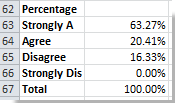You can also show these results as percentages with selecting them and clicking the % (Percent style) in the Number group on the Home tab.

9. Repeat the step 8 to calculate the percentage of each feedback in every question. See screenshot: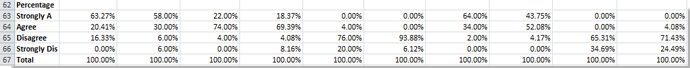####Part 3: Generate a survey report with calculated results above

Now, you can make a survey result report.

10. Select the columns' titles of the survey (A1:K1 in this case), and right click > Copy and then paste them into another blank worksheet by right clicking > Transpose (T). See screenshot: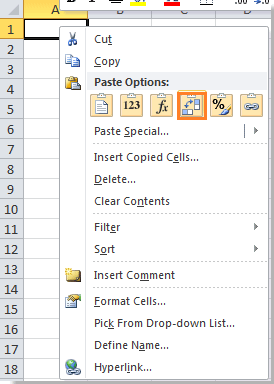If you are using the Microsoft Excel 2007, you can paste these calculated percentages with selecting a blank cell, and then clicking the Home > Paste > Transpose. See the following screenshot: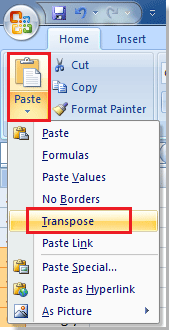11. Edit the title as you need, see screenshot: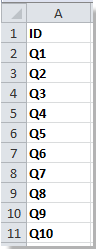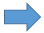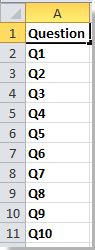12. Select the part you need to display in the report and right click > Copy, and then go to the worksheet you need to paste it and select one blank cell such as Cell B2, a click Home > Paste > Paste Special. See screenshot:13. In the Paste Special dialog, check Values and Transpose in the Paste and Transpose sections, and click OK to close this dialog. See screenshot: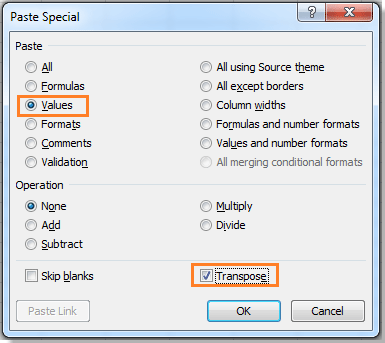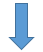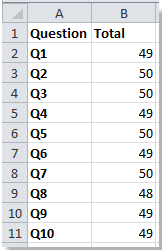Repeat step 10 and 11 to copy and paste the data you need, and then the survey report has been made. See screenshot: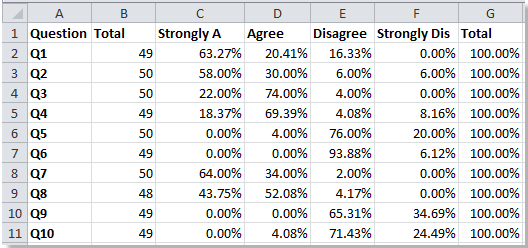No ratings yet. Be the first to rate!
This comment was minimized by the moderator on the site
how to calculate the overall survey results per question when i have % of question
for example 1% agree , 2% disagree ?
This comment was minimized by the moderator on the site
Hi, Nihal, I do not understand your question, do you want to calculate the number of results based on the percentage? For example, total result is 100, and 1% agree, to get the agree number is 1?
This comment was minimized by the moderator on the site
This information was VERY helpful! It gave me exactly the steps needed to analyze the data and then create my charts. Thank you!
This comment was minimized by the moderator on the site
This is really good. I was struggling to sort out my survey results and not that great at Excel but this has been a brilliant help!
This comment was minimized by the moderator on the site
brilliant!! Such a help and easy to understand!!
This comment was minimized by the moderator on the site